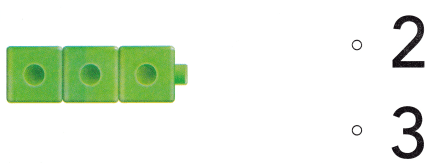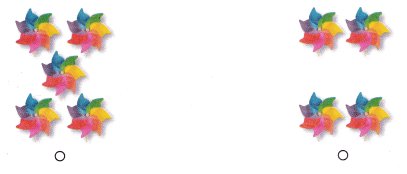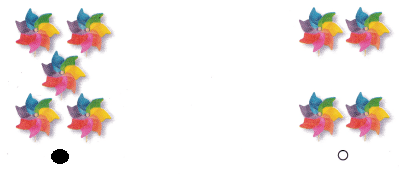# Texas Go Math Kindergarten Lesson 3.4 Answer Key Compare by Matching and Counting Sets to 5

Refer to our Texas Go Math Kindergarten Answer Key Pdf to score good marks in the exams. Test yourself by practicing the problems from Texas Go Math Kindergarten Lesson 3.4 Answer Key Compare by Matching and Counting Sets to 5.

## Texas Go Math Kindergarten Lesson 3.4 Answer Key Compare by Matching and Counting Sets to 5

Unlock the Problem

DIRECTIONS: These are Brandon’s toy cars. How many toy cars does Brandon have? Jay has a number of toy cars that is less than the number of toy cars Brandon has. Use cubes to show how many toy cars Jay might have. Draw the cubes. Write the number. Use matching or counting to compare the sets.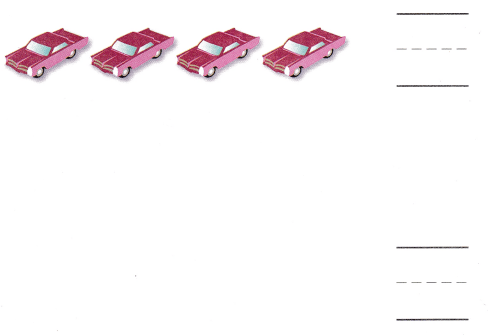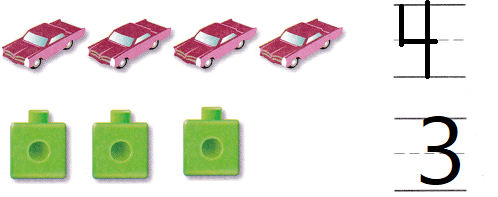Explanation:
Jay has 3 cars now
Drawn the counters as cubes

Try Another Problem

DIRECTIONS: 1. Count how many objects in each set. Write the numbers. Compare the numbers. Circle the number that is greater. 2-3. Count how many objects in each set. Write the numbers. Compare the numbers. Circle the number that is less.

Question 1.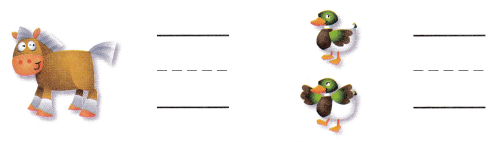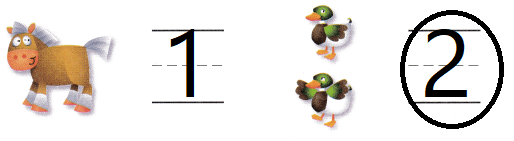Explanation:
Compared the object
circled the number which is more

Question 2.Explanation:
Compared the object
circled the number which is less

Question 3.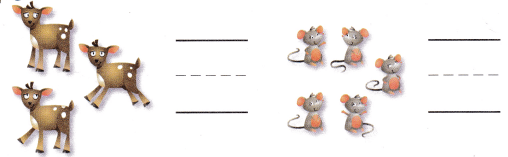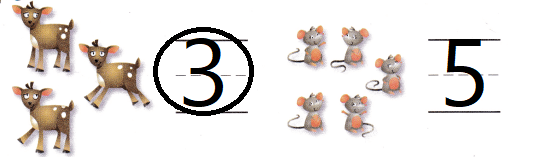Explanation:
Compared the object
circled the number which is less

Share and Show

DIRECTIONS: 4. Kendall has a set of three pencils. Her friend has o set with the same number of pencils. Draw to show the sets of pencils. Compare the sets by matching. Write how many in each set. 5. Draw two sets with different numbers of dots. Show what you know about matching to compare the two sets of dots. Write how many in each set. Circle the greater number.

Question 4.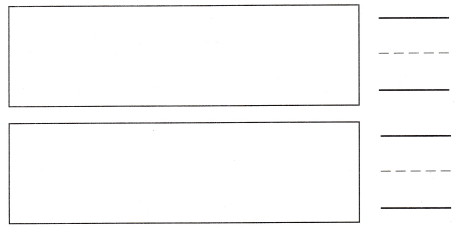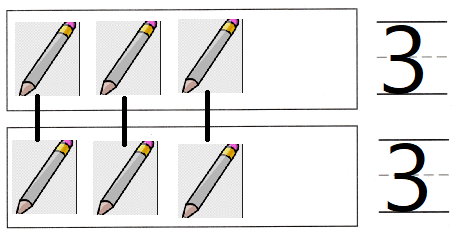Explanation:
Each set has 3 pencils
they are equal.

Question 5.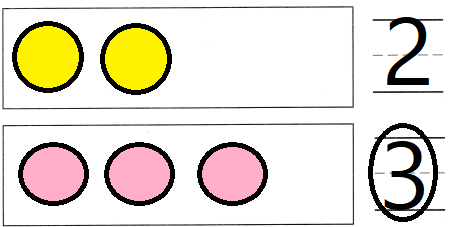Explanation:
Drawn 2 sets with different number of dots
and matched
and circled the greater number.

HOME ACTIVITY • Show your child two sets with a different number of objects in each set. Have him or her use matching to compare the sets.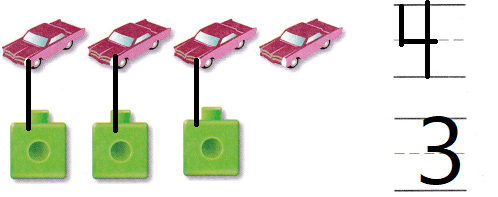Explanation:
Two sets with a different number of objects in each set.
Matched and compared the 2 sets

DIRECTIONS: Choose the correct answer. 6. Which set has a greater number? 7. Which set has the same number as the cube train? 8. Which is less than the number of cubes?

Question 6.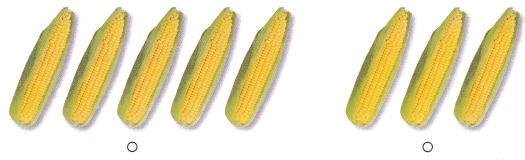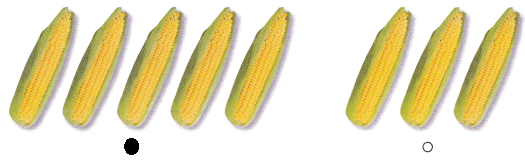Explanation:
Bubbled the option which is greater

Question 7.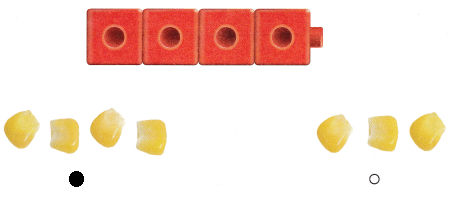Explanation:
Bubbled the option which is equal to the cubes

Question 8.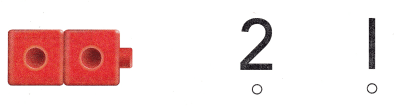Explanation:
Bubbled the option which is less than the cubes

### Texas Go Math Kindergarten Lesson 3.4 Homework and Practice Answer Key

DIRECTIONS: 1. Tori has a set of 4 heart stickers. Her friend has a set with the same number of stickers. Draw to show the sets of stickers. Compare the sets by matching. Write how many in each set. 2. Draw two sets of circles with the same number. Show what you know about matching to compare two sets of objects. Write how many in each set.

Question 1.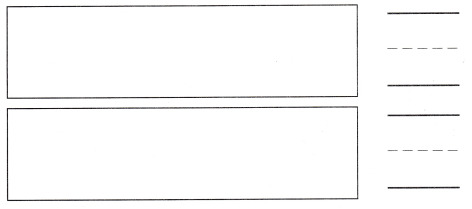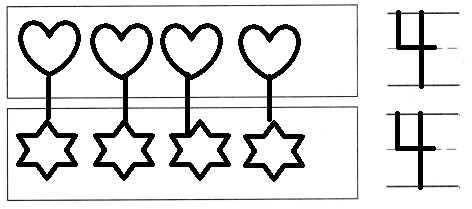Explanation:
Tori has a set of 4 heart stickers.
Her friend has a set with the same number of stickers.
Each has 4 stickers
drawn and compared both

Question 2.Explanation:
Drawn 2 sets of circles
compared and matched
written the number 2

DIRECTIONS: Choose the correct answer. 3. Which is greater than the number of cubes? 4. Which is less than the number of cubes? 5. Which set has a greater number?

Lesson Check

Question 3.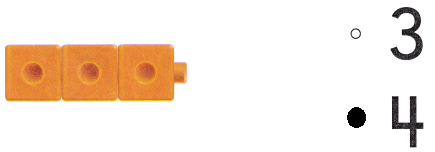Explanation:
Bubbled the number which is greater than the cubes

Question 4.